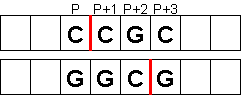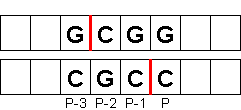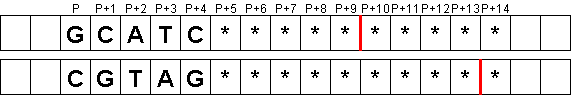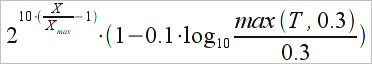JOINProblem Statement
Contest: Marathon Match 70
Problem: RestrictionDigest

### Problem Statement

When a scientist wants to analyze a DNA molecule, they perform a procedure called restriction digest. It uses a set of enzymes which cut the molecule into a certain set of DNA fragments accordingly to certain rules. In this problem you will be given a DNA molecule, and your task will be to construct a set of enzymes which have to be applied to the molecule to produce a set of DNA fragments which satisfy the given restrictions.

### DNA molecule

DNA molecule consists of two strands (chains) of nucleotides, called forward strand and reverse strand. There are only four types of nucleotides - 'A' (Adenine), 'C' (Cytosine), 'G' (Guanine) and 'T' (Thymine); nucleotides A and T are complementary to each other, and so are C and G. The strands consist of N nucleotides each, and for each i between 0 and N-1 the i-th nucleotide of forward strand is complementary to the i-th nucleotide of reverse strand. For example, if the forward strand is "ATGC", then the reverse strand will be "TACG". Thus, the forward strand alone defines the DNA in a unique way.

In this problem the DNA molecule of N nucleotides will be represented as a String dna, consisting of N+1 characters. First N characters will describe the forward strand of the molecule, one character per nucleotide. The last character will be '.' if the molecule is linear, and '+' if it is circular (the ends of the molecule are connected so that the first nucleotide follows the last nucleotide).

### Enzymes

For the purposes of this problem, enzymes are entities which, when applied to a DNA molecule, can cut it into fragments accordingly to certain rules. Each enzyme has a code - a unique String identifier, and a recognition sequence - a String which defines enzyme's behavior. Enzymes' recognition sequences can contain only nucleotide characters ('A', 'C', 'G', 'T') and wildcards (will be explained later). Before introducing the formal rules of enzymes' behavior, let's have a look at several examples. We will assume that the DNA is linear (circular DNA case is similar and will be explained later).

An enzyme with code "AciI" has recognition sequence "CCGC". This enzyme cuts the DNA if its forward strand contains "CCGC" as a contiguous substring (this is called a forward match). Both forward and reverse strands of DNA are cut when a match is found, but generally they are cut in different places. The offsets of the cuts from the start of the match in the forward and the reverse strands are given by two numbers A and B, respectively; for this enzyme A = 1 and B = 3. The cuts done by this enzyme are shown on the image:The more formal way to describe the cuts done by the enzyme in case of a forward match is as follows. Let L denote the length of recognition sequence of the enzyme, and A and B - the offsets of the cuts in forward and reverse strands, respectively. If a forward match is found at position P and includes nucleotides from P to (P+L-1), the cut in the forward strand is done between nucleotides (P+A-1) and (P+A), and the cut in the reverse strand is done between nucleotides (P+B-1) and (P+B). A cut between nucleotides P and (P+1) is considered to be positioned at P.

The enzyme also cuts the DNA if its reverse strand contains the reverse of recognition sequence as a contiguous substring (this is called a reverse match). You can think of it as rotating the molecule 180 degrees and searching for recognition sequence in forward strand of rotated molecule. The same numbers A and B define the offsets of the cuts, but this time in the reverse and the forward strands, respectively. The indices of the nucleotides between which the cuts are done will be the same, if we reverse the indexation of the nucleotides in all DNA (as in rotated molecule); in direct indices the reverse match includes nucleotides from P to (P-L+1), the cut in reverse strand is done between nucleotides (P-A+1) and (P-A), and the cut in forward strand is done between nucleotides (P-B+1) and (P-B).Note that the values of A and B can be zero, negative or greater than L-1; this means that the cuts are done outside of the matched part of the DNA or on its borders (for 0 or L values). However, for a cut to be done, all values of (P+A-1), (P+A), (P+B-1) and (P+B) (for forward matches) or (P-A+1), (P-A), (P-B+1) and (P-B) (for reverse matches) must be valid nucleotides indices, i.e., be between 0 and N-1, inclusive (remember that we consider only linear DNA at the moment; this is different for circular DNA). Thus, for example, enzyme "LweI" has recognition sequence "GCATC", and values of A = 10 and B = 14. Forward and reverse matches for this enzyme will produce the following cuts (nucleotides marked by * can have any value, but must exist in the molecule, while nucleotides marked by . might not exist):Another thing to note is that forward and reverse matches can produce cuts in the same locations; thus, for example, enzyme "EcoRI" has recognition sequence "GAATTC", and values of A = 1 and B = 5. The recognition sequence is complementary to its reverse, so the forward and reverse matches will include the same nucleotides, and the cuts will be done in the same places:#### Wildcards

Recognition patterns can contain wildcards - characters which match any nucleotide from a certain set. The complete list of wildcards and corresponsing sets of matching nucleotides follows.

```Character   Accepted nucleotides
M           A, C
R           A, G
W           A, T
S           C, G
Y           C, T
K           G, T
V           A, C, G
H           A, C, T
D           A, G, T
B           C, G, T
X,N         G, A, T, C```

#### 4-cuts enzymes

Some enzymes do four cuts per match (both forward and reverse match) instead of two. Such enzymes are described with two pairs of offsets (A1, B1) and (A2, B2). Each pair defines two cuts, one in forward strand, and one in reverse strand, just like in the 2-cuts enzymes. All cut positions must be valid for any cuts to be done. Thus, for example, enzyme "BdaI" has recognition sequence "TGANNNNNNTCA", A1 = -10, B1 = -12, A2 = 24, B2 = 22. Each forward match (and each reverse one as well) will result in the following cuts:#### Circular DNA molecules

In circular molecules all nucleotide indices are calculated modulo N. This means that a match can stretch across nucleotides (N-1) and 0, as well as to the left of 0 and/or to the right of (N-1), and still be a valid match. For example, this is a valid forward match for "EcoRI" which starts from nucleotide (N-3) and ends at nucleotide 2:The cuts are also evaluated modulo N; a cut between -2 and -1 is the same as between (N-2) and (N-1), and between (2*N-2) and (2*N-1). This means that all cuts are valid in circular molecule. The cut between nucleotides (N-1) and 0 is considered to be positioned at (N-1).

### Applying a set of enzymes

Applying a given set of enzymes to a given DNA molecule is done as follows. First, for each enzyme from the set, all forward/reverse matches are found, and corresponding cut positions in forward/reverse strands are evaluated (but not performed yet!). After this, all cuts found are simultaneously applied to the DNA; it is possible to have several cuts at the same position, which is equivalent to a single cut at it.

### Input Data

You will be given the following information:
• String dna, describing the given DNA molecule.
• String[] enzymes, describing available types of enzymes. Each element of enzymes will represent a single enzyme and will be formatted as "code seq A B" for 2-cuts enzymes or "code seq A1 B1 A2 B2" for 4-cuts enzymes (here code is enzyme's identifier and seq is its recognition sequence). The contents of enzymes will be the same over all test cases; you can download it here.
• String restrictions, setting restrictions on the task you have to complete. It will always contain 8 elements; the formatting will be explained later.

You have to construct a set of enzymes S which will be applied to the given DNA. Once all cuts are done, each strand of DNA will be separated into several fragments; we are interested in fragments of forward strand only. Let K denote the number of fragments, and L0, L1, ..., LK-1 - the lengths of the fragments sorted in non-ascending order (i.e., Li ≥ Li+1 for all i). The set of enzymes you construct must satisfy all conditions given in restrictions. Here they are:
• restrictions is "minFragmentCountt" (integer), specifying the minimal number of fragments: K ≥ minFragmentCount.
• restrictions is "maxFragmentCount" (integer), specifying the maximal number of fragments: K ≤ maxFragmentCount.
• restrictions is "minFragmentLength" (integer), specifying the minimal length of a fragment: LK-1 ≥ minFragmentLength.
• restrictions is "maxFragmentLength" (integer), specifying the maximal length of a fragment: L0 ≤ maxFragmentLength.
• restrictions is "minFragmentDiff" (floating-point between 0 and 10, inclusive, with exactly 3 digits after decimal point), specifying a restriction on the difference of fragment lengths: 100 * (Li - Li+1) ≥ minFragmentDiff * Li, for each i, 0 ≤ i ≤ K-2.
• restrictions is "minRSLength" (integer), specifying the minimal length of the recognition sequence of each enzyme from set S.
• restrictions is "maxEnzymes" (integer), specifying the maximal number of enzymes in set S.
• restrictions specifies the ranges of positions where at least one cut must be done, and is interpreted as follows. If it is "none", the restriction is simply ignored. Otherwise it is a comma-separated list of pairs "left right", where all lefts and rights are integers, and each pair means that there must be at least one cut positioned from left to right, inclusive. For linear DNA, each pair will satisfy condition 0 ≤ left ≤ right ≤ N-2. For circular DNA, each pair will satisfy condition 0 ≤ left, right ≤ N-1; if left > right, the pair specifies cut positions left, left+1, ..., N-1, 0, 1, ..., right.
Finally, the set S of enzymes must be irreducible: for any set S' obtained from S by removing one enzyme, the sets of cuts (in the forward strand) produced in this DNA by sets S and S' must be different.

### Return value

Your program must find and return as many distinct sets S which satisfy the given restrictions as possible. If you can find more than a hundred of such sets, return any 100 of them. The return value is a String[], in which each element describes one set as a space-separated list of 0-based enzyme indices in enzymes array, sorted in strictly ascending order (without duplicates), written without extra leading zeroes.

### Scoring

The individual score for a test case is calculated as follows. Invalid return of any kind results in 0 score. If the return is valid, the score will be X + T/100.0, where X is the number of sets S you have found, and T is the processor time used by your program (in seconds).

Your overall score will be calculated as sum of normalized scores over all test cases. Normalized score for a valid return is given with the following formulawhere Xmax is the maximal number of sets found by any competitor for this test case. There are two exceptions from the general formula. If Xmax is 0, then all competitors get 0 normalized score for this test case. If X is 0, the corresponding competitor gets 0 normalized score for this test case.

### Test Case Generation

The test cases will be generated as follows. enzymes is a constant array taken from this file.

dna is either taken from a real molecula, or generated using Markov chain model. DNA length is chosen uniformly between 5000 and 100000, inclusive. 11 samples of real DNA molecules were analyzed to produce probabilities of all possible sequences of 1..4 nucleotides appearing in the molecula. The resulted distributions can be downloaded here.

When generating a DNA, the distribution number is first chosen. It will be distribution 11 with probability 1/3 and one of distributions 1-10 with probability 1/15 each. Then dna is constructed character by character based on the chosen distribution. At east step, last 3 characters are considered (let them form a string prev) and the next character is chosen as next with probability proportional to the number of occurrences of nucleotide sequence prev+next in the chosen distribution. The first 3 characters are generated based on previous 0, 1 and 2 characters, correspondingly. The last character of dna, which specifies whether it is linear or circular, is chosen as '.' or '+' with equal probabilities.

restrictions are generated in a rather complicated manner.
• minFragmentCount and maxFragmentCount are chosen uniformly between 3 and 15, inclusive, so that maxFragmentCount ≥ minFragmentCount.
• minFragmentLength, maxFragmentLength and minFragmentDiff are chosen together in the following way. minFragmentLength is chosen uniformly between 1 and N / minFragmentCount, inclusive. maxFragmentLength is chosen as ceil(N / maxFragmentCount) / x, where x is chosen uniformly between 0 and 1, so that maxFragmentLength ≤ N. If after this minFragmentLength > maxFragmentLength, generation of these three parameters restarts. Otherwise minFragmentDiff is chosen uniformly between 0 (inclusive) and 10 (exclusive), and it is verified that there exists a sequence of fragment lengths which satisfies the first 5 restrictions for values minFragmentCount, maxFragmentCount, minFragmentLength, maxFragmentLength, 10*minFragmentDiff (note that each sequence that satisfies the 5-th restriction (1-based) for 10*minFragmentDiff will also satisfy it for minFragmentDiff, but not vice versa); if it doesn't, generation restarts. Note that verification is done only for fragment lengths, it is not verified that there exists a set of enzymes that would produce exactly these fragment lengths.
• minRSLength is chosen uniformly between minRS and (maxRS+minRS)/2, inclusive, where minRS and maxRS are minimal and maximal length of recognition sequence of all enzymes present in the input.
• maxEnzymes is chosen uniformly between maxFragmentCount/2 and maxFragmentCount, inclusive.
• ranges are present with probability 1/2; if they are, the number of ranges in the restriction is chosen uniformly between 1 and 3, inclusive, each pair is gerenated independently, and left and right of each range are chosen uniformly so that they form a valid range (see definition of ranges).

### Tester

A tester is available for offline testing. You can check its source code for a precise implementation of test case generation and scoring. Note that it provides only test cases with generated DNA molecules.

### Prizes and conditions

In order to receive the prize money, you will need to provide an explanation of how your solution works. Note that it should not be submitted anywhere during the coding phase. Instead, if you win a prize, a TopCoder representative will contact you directly in order to collect it.

### Definition

 Class: RestrictionDigest Method: findEnzymeCocktail Parameters: String, String[], String[] Returns: String[] Method signature: String[] findEnzymeCocktail(String dna, String[] enzymes, String[] restrictions) (be sure your method is public)

### Notes

-The memory limit is 1024 MB and the time limit is 30 seconds (which includes only time spent in your code).
-There is no explicit code size limit. The implicit source code size limit is around 1 MB (it is not advisable to submit codes of size close to that or larger). Once your code is compiled, the binary size should not exceed 1 MB.
-The compilation time limit is 30 seconds. You can find information about compilers that we use and compilation options here.
-There are 10 example test cases. First 9 examples have seeds 1..9, the last example uses a real DNA (distribution 11).
-There are 100 full submission test cases. Of them, 10 test cases use real DNA (9 cases from distribution 11 and 1 case from distribution 1), the rest are generated artificially.
-99 system test cases will use real DNAs, the rest will be generated artificially. 90 real test cases will come from distribution 11 and 9 test cases will come from distributions 2-10 (1 test case per distribution).

### Constraints

-All numbers in restrictions are given without leading zeroes.

### Examples

0)

```seed = 1
```
 ```Model = 3 DNA length = 93936 Circular Restrictions: 4 6 4585 88190 0.785 3 3 78628 3822,29206 2141,29245 91617```
1)

```seed = 2
```
 ```Model = 10 DNA length = 64970 Linear Restrictions: 8 12 3935 53236 1.370 3 12 16479 25185,34185 55960```
2)

```seed = 3
```
 ```Model = 6 DNA length = 44947 Linear Restrictions: 6 11 2397 5733 0.355 6 10 none```
3)

```seed = 4
```
 ```Model = 10 DNA length = 73955 Circular Restrictions: 4 5 3133 40608 1.538 4 4 none```
4)

```seed = 5
```
 ```Model = 7 DNA length = 58643 Circular Restrictions: 6 11 1348 20746 1.099 3 11 42688 44203```
5)

```seed = 6
```
 ```Model = 10 DNA length = 71837 Linear Restrictions: 5 6 13277 34830 0.188 3 4 none``` We suspect that this test case has no solutions.
6)

```seed = 7
```
 ```Model = 10 DNA length = 96850 Linear Restrictions: 5 8 424 81315 1.836 6 7 none```
7)

```seed = 8
```
 ```Model = 4 DNA length = 39919 Linear Restrictions: 6 15 2989 13517 1.113 7 9 none```
8)

```seed = 9
```
 ```Model = 5 DNA length = 25094 Circular Restrictions: 8 9 720 10306 1.677 6 6 none```
9)

```seed = 10 real10
```
 This test case uses real DNA (distribution 10).

This problem statement is the exclusive and proprietary property of TopCoder, Inc. Any unauthorized use or reproduction of this information without the prior written consent of TopCoder, Inc. is strictly prohibited. (c)2010, TopCoder, Inc. All rights reserved.